﻿ Linear Inequalities in Two variables
LINEAR INEQUALITIES IN TWO VARIABLES

Here's what this lesson offers:

## Going from Linear Equations to Linear Inequalities

 You've already learned that the graph of $\,y = x + 1\,$ is the line shown at right. This line is the picture of all the points $\,(x,y)\,$ that make the equation ‘$\,y=x+1\,$’ true. How can ‘$\,y=x+1\,$’ be true? For a given $\,x$-value, the $\,y$-value must equal $\,x + 1\,$. For each $\,x$-value, there is exactly one corresponding $\,y$-value—whatever $\,x\,$ is, plus $\,1\,$. The line is the picture of all the points $\,(x,x+1)\,$, as $\,x\,$ varies over all real numbers: $$\text{the line ‘\,y=x+1\,’ is all points of the form:} \qquad (\ \ x\ \ ,\overbrace{x+1}^{\text{the y-value EQUALS x+1}})$$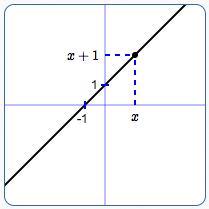graph of $\,y = x + 1\,$: all points of the form $\,(x,x+1)$

Question:
What happens if the verb in the sentence ‘$\,y \color{red}{=} x+1\,$’ is changed from ‘$\,\color{red}{=}\,$’ to $\,\lt\,$, $\,\gt\,$, $\,\le\,$, or $\,\ge\,$?

You go from a linear equation in two variables, to a linear inequality in two variables.
The solution set changes dramatically!
What was a line now becomes an entire half-plane:graph of $\,y < x + 1\,$: all points of the form $\,(x,y)$ where the $y$-value is less than $\,x+1\,$ line is dashed; shade below the linegraph of $\,y > x + 1\,$: all points of the form $\,(x,y)$ where the $y$-value is greater than $\,x+1\,$ line is dashed; shade above the linegraph of $\,y \le x + 1\,$: all points of the form $\,(x,y)$ where the $y$-value is less than or equal to $\,x+1\,$ line is solid; also shade below the line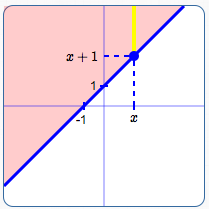graph of $\,y \ge x + 1\,$: all points of the form $\,(x,y)$ where the $y$-value is greater than or equal to $\,x+1\,$ line is solid; also shade above the line

## Important Concepts for Graphing Linear Inequalities in Two Variables

• DEFINITION: LINEAR INEQUALITY IN TWO VARIABLES
A linear inequality in two variables is a sentence of the form $$ax + by + c < 0\,,$$ where $\,a\,$ and $\,b\,$ are not both zero; $\,c\,$ can be any real number.
The inequality symbol can be any of these: $\,\lt\,$, $\,\gt\,$, $\,\le\,$, or $\,\ge\,$
Remember:    a ‘sentence of the form ...’    really means    a ‘sentence that can be put in the form ...’
• EXAMPLES OF LINEAR INEQUALITIES IN TWO VARIABLES

 $3x - 4y + 5 > 0$ $y \le 5x - 1$ $x \ge 2\,$(shorthand for $\,x + 0y \ge 2\,$) $y < 5\,$(shorthand for $\,0x + y < 5\,$

Key ideas for recognizing linear inequalities in two variables:
• the verb must be an inequality symbol: $\,\lt\,$, $\,\le\,$, $\,\gt\,$, or $\,\ge\,$
• the variables must be raised only to the first power :
no squares, no variables in denominators, no variables under square roots, and so on
• you don't need to have both $\,x\,$ and $\,y\,$, but you must have at least one of these variables
• LINEAR INEQUALITIES GRAPH AS HALF-PLANES
Every linear inequality in two variables graphs as a half-plane:
• if the verb is $\,\lt\,$ or $\,\gt\,$, the boundary line is not included (dashed)
• if the verb is $\,\le\,$ or $\,\ge\,$, the boundary line is included (solid)
If the linear inequality is in slope-intercept form (like $\,y < mx + b\,$), then it's easy to know which half of the line to shade:
• if the sentence is   $\,y \lt mx + b\,$   or   $\,y \le mx + b\,$, shade BELOW the line
• if the sentence is   $\,y \gt mx + b\,$   or   $\,y \ge mx + b\,$, shade ABOVE the line
This only works if the inequality is in slope-intercept form!
Of course, you can always put a sentence in slope-intercept form, by solving for $\,y\,$.
Then, you can use this method.
But, the ‘test point method’ (below) is usually quicker-and-easier, if the sentence isn't already in slope-intercept form.

## The Test Point Method for Graphing Linear Inequalities in Two Variables

 So, what about graphing something like $\,2x - y < 3\,$, which isn't in slope-intercept form? You can (if desired) solve for $\,y\,$, and then use the method above: $$\begin{gather} 2x - y < 3\cr -y < -2x + 3\cr y > 2x - 3 \end{gather}$$ (Remember to change the direction of the inequality symbol when you multiply/divide by a negative number.) The graph of $\,2x - y < 3\,$ is the same as the graph of $\,y > 2x - 3\,$. Graph the line $\,y = 2x - 3\,$ (dashed), and then shade everything above (see right). However, there's an easier way. Keep reading!graph of $\,2x - y < 3\,$ (which is equivalent to $\,y > 2x - 3\,$)

The ‘Test Point Method’ is so-called because it involves choosing a ‘test point’ to decide which side of the line to shade.
The process is illustrated with an example: graphing $\,2x - y < 3\,$.
The Test point Method is usually easiest to use with sentences that aren't in slope-intercept form.

GRAPH, USING THE TEST POINT METHOD: $\,2x - y < 3$
 Step 1:   IDENTIFICATION Recognize that ‘$\,2x - y < 3\,$’ is a linear inequality in two variables. Therefore, you know the graph is a half-plane. You need the boundary line; you need to know which side to shade. Step 2:   BOUNDARY LINE Graph the boundary line $\,2x - y = 3\,$ using the intercept method. When $\,x = 0\,$, $\,y = -3\,$. When $\,y = 0\,$, $\,x = \frac{3}{2}\,$. Since the verb in ‘$\,2x - y < 3\,$’ is ‘$\,<\,$’, this line is not included in the solution set. Therefore, the line is dashed.graph the boundary lineusing the intercept method Step 3:   TEST POINT TO DECIDE WHICH SIDE TO SHADE Choose a simple point that is NOT on the line. Whenever $\,(0,0)\,$ is available, choose it! Zeroes are very easy to work with. Is $\,(0,0)\,$ in the solution set? Substitute $\,x = 0\,$ and $\,y = 0\,$ into the original sentence ($\,2x - y < 3\,$), to see if it is true or false. Put a question mark over the inequality symbol, since you're asking a question: $$2(0) - 0 \overset{?}{<} 3$$ If the result is TRUE, shade the side containing the test point. If the result is FALSE, shade the other side. Since ‘$\,0 < 3\,$’ is TRUE, shade the side containing $\,(0,0)\,$. Done! With so many zeroes involved in this method,computations can often be done in your head, making this QUICK and EASY!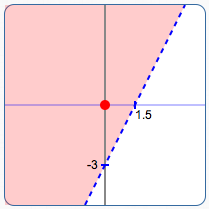choose test point $\,(0,0)\,$: since ‘$2(0) - 0 < 3\,$’ is TRUE, shade the side containing the test point

## Special Linear Inequalities in Two Variables: You only see one variable

Out of context, sentences like ‘$\,x \ge 2\,$’ and ‘$\,y < 5\,$’ can be confusing.
You only see one variable, but that doesn't necessarily mean that there isn't another variable with a zero coefficient!

Out of context, here are clues to what is likely wanted:

• ‘Solve: $\,x\ge 2\,$’ probably wants you to treat this as an inequality in ONE variable.
• ‘Graph: $\,x\ge 2\,$’ probably wants you to treat this as an inequality in TWO variables.
As shown below, there is a BIG difference in the nature of the solution set!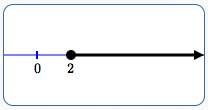graph of $\,x\ge 2\,$, viewed as an inequality in ONE variable Viewed as an inequality in one variable, the solution set of ‘$\,x\ge 2\,$’ is the set of all numbers that are greater than or equal to $\,2\,$. The solution set is the interval $\,[2,\infty)\,$, shown at left.graph of $\,x\ge 2\,$, viewed as an inequality in TWO variables Viewed as an inequality in two variables, ‘$\,x\ge 2\,$’ is really a shorthand for ‘$\,x + 0y \ge 2\,$’. The solution set is the set of all points $\,(x,y)\,$, where the $x$-value is greater than or equal to $\,2\,$. The $y$-value can be anything! Here are examples of substitution into ‘$\,x + 0y \ge 2\,$’: The point $\,(2,5)\,$ is in the solution set, since ‘$\,2 + 0(5) \ge 2\,$’ is TRUE. The point $\,(3.5,-7.4)\,$ is in the solution set, since ‘$\,3.5 + 0(-7.4) \ge 2\,$’ is TRUE. The graph is the half-plane shown at left. This is the picture of all the points with $x$-value greater than or equal to $\,2\,$.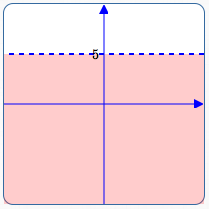graph of $\,y\lt 5\,$, viewed as an inequality in TWO variables Viewed as an inequality in two variables, ‘$\,y\lt 5\,$’ is really a shorthand for ‘$\,0x + y \lt 5\,$’. The solution set is the set of all points $\,(x,y)\,$, where the $y$-value is less than $\,5\,$. The $x$-value can be anything! Here are examples of substitution into ‘$\,0x + y \lt 5\,$’: The point $\,(2,4)\,$ is in the solution set, since ‘$\,0(2) + 4 \lt 5\,$’ is TRUE. The point $\,(-7.4,-3)\,$ is in the solution set, since ‘$\,0(-7.4) -3 \lt 5\,$’ is TRUE. The graph is the half-plane shown at left. This is the picture of all the points with $y$-value less than $\,5\,$.
Master the ideas from this section